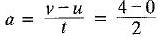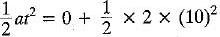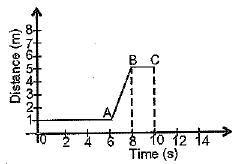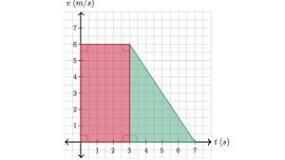Courses

# MCQ : Motion - 2

## 10 Questions MCQ Test Science Class 9 | MCQ : Motion - 2

Description
This mock test of MCQ : Motion - 2 for Class 9 helps you for every Class 9 entrance exam. This contains 10 Multiple Choice Questions for Class 9 MCQ : Motion - 2 (mcq) to study with solutions a complete question bank. The solved questions answers in this MCQ : Motion - 2 quiz give you a good mix of easy questions and tough questions. Class 9 students definitely take this MCQ : Motion - 2 exercise for a better result in the exam. You can find other MCQ : Motion - 2 extra questions, long questions & short questions for Class 9 on EduRev as well by searching above.
QUESTION: 1

### Equations of motion can be used for a body having

Solution:

A body under constant acceleration is uniformly accelerated motion. This is when a constant external force is applied to the body. The direction of acceleration is very important in the change of velocity. However, the direction of the accelerration of a body may not be the same as the direction of its motion.

QUESTION: 2

### The magnitude of the displacement of an object is always

Solution:

Displacement is the shortest straight line distance between initial and final position . This means that there doesn't exist any other path which is shorter than it .
If the object travels with that shortest straight line path then the magnitude of distance and displacement would be same . If the object doesn't do so and travels with any other paths then as the other path is not the shortest path , so it will be larger than that of displacement . So if the object travels with this path then the magnitude of displacement will be smaller than the distance.

Thus , the magnitude of displacement may be equal or smaller than that of distance.

QUESTION: 3

### For a body starting from rest, the displacement in 10 seconds, when it acquires 4 ms-1 in 2 seconds is

Solution:

v = u + at
or= 2m/s2
s = ut += 100 m,

QUESTION: 4

Retardation of a body is expressed in

Solution:

Retardation, actually, is acceleration that acts opposite to the direction of motion of an object. So, under retardation, an object accelerates away from its direction of motion, so it's velocity in that direction decreases. The SI unit of retardation, it being just an oppositely directed acceleration, is m/s2.

QUESTION: 5

The v-t graph for the x-t graph given here is best drawn asSolution:
QUESTION: 6

On a velocity-time graph, a horizontal straight line corresponds to motion at

Solution:

it is horizontal so, angle is zero and also accleration is zero. Hence constant velocity.

QUESTION: 7

For a uniformly accelerated body with initial and final velocities as u and v ms-1, the average velocity

Solution:

Average velocity  of an object is equal to its final velocity plus initial velocity, divided by 2.
Therefore, the answer is, (u + v) / 2

QUESTION: 8

Which of the following graphs show that the body is at rest?

Solution:

The correct option is Option A.

In figure A, we can see that the object continues to remain at a fixed place while the time keeps running. So, the object is at rest, as because the object does not change it's position with time.

QUESTION: 9

Area below v - t graph is a measure of

Solution:

"Displacement" is the quantity which is measured by the area occupied under the velocity time graph distance. Moving something or someone from its or his or her place and position is called displacement.

QUESTION: 10

The slope of velocity-time graph gives

Solution:The slope of a velocity graph represents the acceleration of the object. So, the value of the slope at a particular time represents the acceleration of the object at that instant.